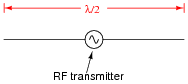## AC Electric Circuits

• #### Question 1

What does the acronym RF stand form, in reference to radio-related electronics?

• #### Question 2

We know at this point that any circuit comprised of inductance (L) and capacitance (C) is capable of resonating: attaining large values of AC voltage and current if “excited” at the proper frequency. The so-called tank circuit is the simplest example of this:The less resistance (R) such a circuit has, the better its ability to resonate.

We also know that any piece of wire contains both inductance and capacitance, distributed along its length. These properties are not necessarily intentional - they exist whether we would want them to or not: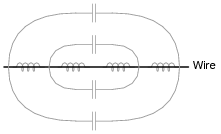Given that the electrical resistance of a continuous piece of metal wire is usually quite low, describe what these natural properties of inductance and capacitance mean with regard to that wire’s function as an electrical element.

• #### Question 3

Shown here is a simple quarter-wave antenna, comprised of a single wire projecting vertically from one terminal of an RF voltage source, the other terminal connected to earth ground:Re-draw this illustration, showing the equivalent inductance and capacitance exhibited by this antenna. Show these properties using actual inductor and capacitor symbols.

• #### Question 4

Shown here is a simple dipole antenna, comprised of two equal-length wires projecting from the terminals of an RF voltage source: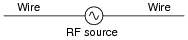Re-draw this illustration, showing the equivalent inductance and capacitance exhibited by this antenna. Show these properties using actual inductor and capacitor symbols.

• #### Question 5

A Scottish physicist named James Clerk Maxwell made an astonishing theoretical prediction in the nineteenth century, which he expressed with these two equations:

$$\oint E . dl = - \frac{d\Phi B}{dt}$$

$$\oint B \ . \ dl = \mu_0I+\mu_0\epsilon_0 \frac{d\Phi _E}{dt}$$

The first equation states that an electric field (E) will be produced in open space by a changing magnetic flux $$\frac{d\Phi B}{dt}$$. The second equation states than a magnetic field (B) will be produced in open space either by an electric current (I) or by a changing electric flux ([(d ΦE)/dt]). Given this complementary relationship, Maxwell reasoned, it was possible for a changing electric field to create a changing magnetic field which would then create another changing electric field, and so on. This cause-and-effect cycle could continue, ad infinitum, with fast-changing electric and magnetic fields radiating off into open space without needing wires to carry or guide them. In other words, the complementary fields would be self-sustaining as they traveled.

Explain the significance of Maxwell’s prediction, especially as it relates to electronics.

• #### Question 6

In 1887, a German physicist named Heinrich Hertz successfully demonstrated the existence of electromagnetic waves. Examine the following schematic of the apparatus he used to do this, and explain what significance Hertz’s discovery has to do with your study of electronics: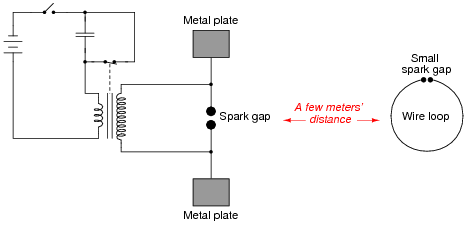• #### Question 7

Given James Clerk Maxwell’s prediction of electromagnetic waves arising from the self-sustenance of changing electric and magnetic fields in open space, what sort of a device or collection of devices do you think we would need to create electromagnetic waves oscillating at a frequency within the range attainable by an electric circuit? In other words, what kind of component(s) would we attach to a source of high-frequency AC to radiate these waves?

• #### Question 8

Although radio transmitter antennae ideally possesses only inductance and capacitance (no resistance), in practice they are found to be very dissipative. In other words, they tend to act as large resistors to the transmitters they are connected to. Explain why this is. In what form is the dissipated energy manifest (heat, light, or something else)?

• #### Question 9

A crystal goblet may be shattered if exposed to high-intensity sound. Less volume is required to shatter the goblet if the sound is at such a frequency that it resonates with the goblet’s natural frequency. That is, there will be maximum transfer of energy to the goblet if the sound waves are transmitted at precisely the goblet’s resonant frequency.

How does this phenomenon relate to the reception of radio waves, since we know that a radio antenna effectively acts as a resonant LC (inductance/capacitance) network?

• #### Question 10

Radio waves are comprised of oscillating electric and magnetic fields, which radiate away from sources of high-frequency AC at (nearly) the speed of light. An important measure of a radio wave is its wavelength, defined as the distance the wave travels in one complete cycle.

Suppose a radio transmitter operates at a fixed frequency of 950 kHz. Calculate the approximate wavelength (λ) of the radio waves emanating from the transmitter tower, in the metric distance unit of meters. Also, write the equation you used to solve for λ.

• #### Question 11

A radio antenna possesses both parasitic capacitance and distributed inductance, distributed along its entire length: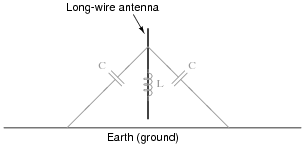Ideally, an antenna only exhibits these electrical properties, with no resistance. What does this suggest about the electrical behavior of an antenna, especially compared to the behavior of other LC circuits you are familiar with?

• #### Question 12

When performing tests on a radio transmitter, it is often necessary to do so without actually broadcasting a signal through an antenna. In such scenarios, an equivalent resistor is connected to the output of the transmitter instead of an actual antenna. If chosen properly, the resistor “looks” the same as an antenna from the perspective of the transmitter.

Explain how this is possible, since real antennae are built to have as little resistance as possible. How can a resistor adequately substitute for an antenna, which is nothing like a resistor in either construction or purpose?